• c代码实现哈希表线性探测再散列。关键字均为纯数字。查找时为单次查找，未加入循环
• 哈希表-线性探测法理论 线性探测法的理论我们在上一篇博客已经阐述了。 现在我们来看看线性探测法的增删查的代码思想： 哈希表的增加元素： 注意：往后遍历寻找空闲位置的时候，要注意是环形遍历哦！不然访问数组...
哈希表-线性探测法理论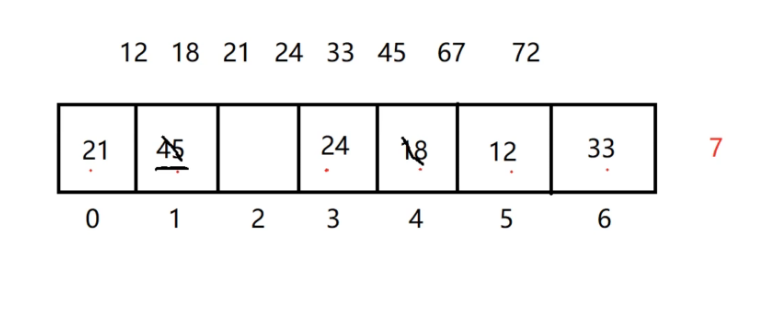线性探测法的理论我们在上一篇博客已经阐述了。 现在我们来看看线性探测法的增删查的代码思想：
哈希表的增加元素：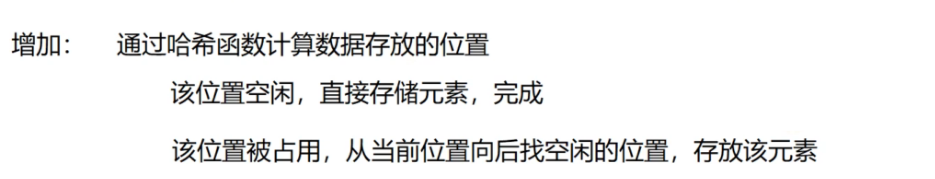注意：往后遍历寻找空闲位置的时候，要注意是环形遍历哦！不然访问数组就越界了。 我们还要注意：在添加元素，发生位置被占用，即发生哈希冲突后，在向后遍历寻找空闲位置的时候，我们要知道，这个空闲的位置是有两种情况的：1、这个位置一直是空的，没放过元素。2、这个位置是空的，以前放过元素，后来被删除了。
哈希表的查询操作：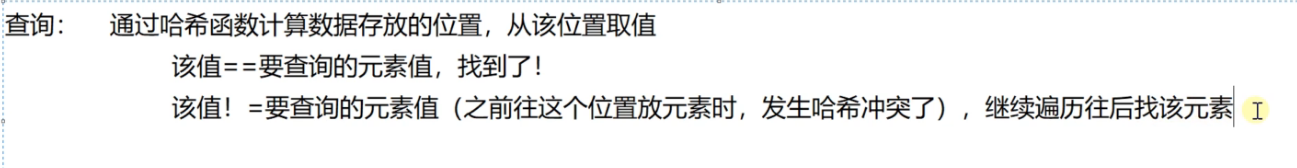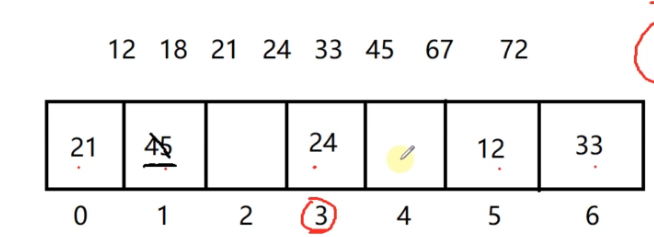当用哈希函数计算得出的下标值是3，然后去访问数组，查询时，发现该值不等于要查询的元素的值val，说明当时放val的时候发生了哈希冲突，这时候就要向后遍历了，访问4下标的时候发现这个位置是空的，如果这个位置一直是空的，则就不用继续向后找了，val不存在！因为是线性探测法，所以当时val如果要放的时候肯定是要放在这里的。但是如果这个位置是空的，但是之前放过元素，后来被删除了，这个位置之前存放了元素，然后val插入的时候，就插到后面的空闲的位置了，所以此时我们还要继续往后遍历寻找val值。所以我们需要定义一个Bucket节点来表示每一个元素的所有的内容。
//桶的状态
enum State
{
STATE_UNUSE, //从未使用过的桶
STATE_USING, //正在使用的桶 放着是一个有效的元素，没有被删过
STATE_DEL,  //元素被删除了的桶，认为桶里的元素无效了
};
//我们删除桶里的元素，并不是真正把值删除掉，而是把桶的状态置为STATE_DEL就认为桶里的元素无效了
//桶的类型
struct Bucket
{
Bucket(int key = 0, State state = STATE_UNUSE)
: key_(key)
, state_(state)
{}

int key_;      //存储的数据
State state_;  //桶的当前状态
};哈希表删除的操作：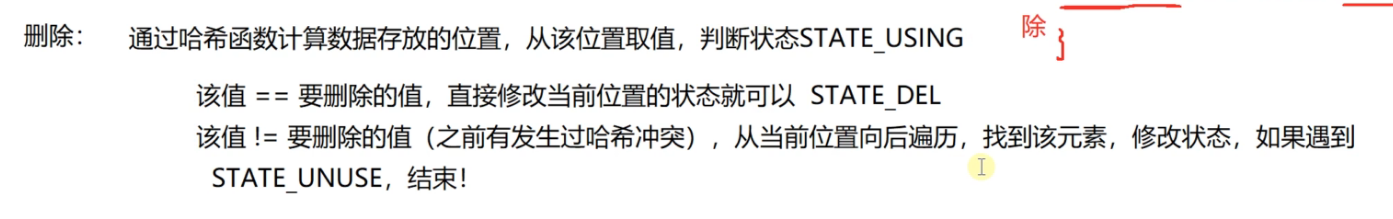哈希表-线性探测法代码实现
#include <iostream>
using namespace std;

//桶的状态
enum State
{
STATE_UNUSE, //从未使用过的桶
STATE_USING, //正在使用的桶 放着是一个有效的元素，没有被删过
STATE_DEL,  //元素被删除了的桶，认为桶里的元素无效了
};
//我们删除桶里的元素，并不是真正把值删除掉，而是把桶的状态置为STATE_DEL就认为桶里的元素无效了

//桶的类型
struct Bucket
{
Bucket(int key = 0, State state = STATE_UNUSE)
: key_(key)
, state_(state)
{}

int key_;      //存储的数据
State state_;  //桶的当前状态
};

//线性探测哈希表类型
class HashTable
{
public:
HashTable(int size = primes_, double loadFactor = 0.75)//构造函数
: useBucketNum_(0)
, primeIdx_(0)
{
//把用户传入的size调整到最近的比较大的素数上
if (size != primes_)
{
for (; primeIdx_ < PRIME_SIZE; primeIdx_++)
{
if (primes_[primeIdx_] >= size)
break;
}
//用户传入的size值过大，已经超过最后一个素数，调整到最后一个素数
if (primeIdx_ == PRIME_SIZE)
{
primeIdx_--;
}
}

tableSize_ = primes_[primeIdx_];
table_ = new Bucket[tableSize_];//开辟构造，调用Bucket的默认构造函数初始化
}

~HashTable()//析构函数
{
delete[]table_;
table_ = nullptr;
}

public:
//插入元素
bool insert(int key)
{
//考虑扩容
double factor = useBucketNum_*1.0 / tableSize_;
cout << "factor:" << factor << endl;
{
//哈希表开始扩容
expand();
}

int idx = key % tableSize_;//计算下标

int i = idx;
do
{
if (table_[i].state_ != STATE_USING)
{
table_[i].state_ = STATE_USING;
table_[i].key_ = key;
useBucketNum_++;
return true;//O(1)
}
i = (i + 1) % tableSize_;//环形路径
} while (i != idx);//O(n)
//最多跑一圈，而且不可能跑一圈，因为哈希表是不可能放满的，超过加载因子就扩容
//do while 是先执行一次，再判断循环
return false;
}

//删除元素，只是把当前状态修改一下而已
bool erase(int key)
{
int idx = key % tableSize_;//计算出哈希值

int i = idx;
do
{
if (table_[i].state_ == STATE_USING && table_[i].key_ == key)
{
table_[i].state_ = STATE_DEL;
useBucketNum_--;
}
i = (i + 1) % tableSize_;
} while (table_[i].state_ != STATE_UNUSE && i != idx);

return true;
}

//查询  count(key)
bool find(int key)
{
int idx = key % tableSize_;

int i = idx;
do
{
if (table_[i].state_ == STATE_USING && table_[i].key_ == key)
{
return true;
}
i = (i + 1) % tableSize_;
} while (table_[i].state_ != STATE_UNUSE && i != idx);

return false;
}

private:
//扩容操作
void expand()
{
++primeIdx_;
if (primeIdx_ == PRIME_SIZE)//越界了
{
throw "HashTable is too large, can not expand anymore!";
}

Bucket* newTable = new Bucket[primes_[primeIdx_]];
for (int i = 0; i < tableSize_; i++)
{
if (table_[i].state_ == STATE_USING)//旧表有效的数据，重新哈希放到扩容后的新表
{
int idx = table_[i].key_ % primes_[primeIdx_];

int k = idx;
do
{
if (newTable[k].state_ != STATE_USING)//空闲的
{
newTable[k].state_ = STATE_USING;
newTable[k].key_ = table_[i].key_;
break;
}
k = (k + 1) % primes_[primeIdx_];
} while (k != idx);
}
}

delete[]table_;
table_ = newTable;
tableSize_ = primes_[primeIdx_];
}

private:
Bucket* table_;//指向动态开辟的哈希表，不要使用vector，因为它是二倍自动扩容，自增长
//要用vector也可以，因为哈希表有装载因子，达到0.75，哈希表进行扩容
int tableSize_;//哈希表当前的长度
int useBucketNum_;//已经使用的桶的个数

static const int PRIME_SIZE = 10;//素数表的大小
static int primes_[PRIME_SIZE];//素数表
int primeIdx_;//当前使用的素数在素数表中的下标值
};

int HashTable::primes_[PRIME_SIZE] = { 3, 7, 23, 47, 97, 251, 443, 911, 1471, 42773 };//素数表的定义
//素数表的最后几个元素代表开辟哈希表数组堆空间的最大的一些内存大小，再大的话堆内存就开辟失败了
//所有哈希表的素数表都是一样的，可以定义成静态的

int main()
{
HashTable htable;
htable.insert(21);
htable.insert(32);
htable.insert(14);
htable.insert(15);

htable.insert(22);

cout << htable.find(21) << endl;
htable.erase(21);
cout << htable.find(21) << endl;
}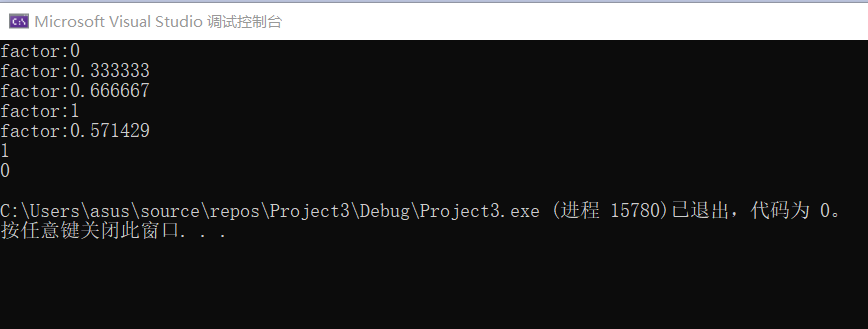展开全文算法
• NULL 博文链接：https://128kj.iteye.com/blog/1744810
• //使用线性探索处理冲突 { int n = max_ ( m ) ; int k , H ; for ( int i = 0 ; i < p ; i ++ ) { cin >> H ; k = H % n ; if ( hash [ k ] . key == 0 ) hash [ k ] . key = H ; ...
#include<iostream>
using namespace std;
#define m 30 //表长
#define ERROR -1
typedef struct key
{
int key;
}Hashtable;
void chushi(Hashtable hash[])
{
for(int i=0;i<m;i++)
{
hash[i].key=0;
}
}
int max_(int n)//取出不大于m的最大素数；
{
int i;
for(int j=m;j>1;j--)
{
for(i=2;i<j/2;i++)
{
if(j%i==0)
break;
}
if(i==j/2)
return j;
}
}
void CreateHash(Hashtable hash [],int p)//使用线性探索法处理冲突
{
int n=max_(m);
int k,H;
for(int i=0;i<p;i++)
{
cin>>H;
k=H%n;
if(hash[k].key==0)
hash[k].key=H;
else
{
for(int j=1;j<m;++j)
{
k=(k+j)%m;
if(hash[k].key==0)
{
hash[k].key=H;
break;
}

}
}
}
}
void output(Hashtable hash [])
{
for(int i=0;i<m;i++)
{
cout<<hash[i].key<<" ";
}
cout<<endl;
}
int FindHash(Hashtable hash [],int H)
{
int n=max_(m);
int k;
for(int i=0;i<m;i++)
{
k=H%n;
if(hash[k].key==H)
return k;
else
{
for(int j=1;j<=i;j++)
{
k=(k+j)%m;
if(hash[k].key==H)
return k;
}
}
}
return -1;
}
int main()
{
int p;
cout<<"输入数据个数:";
cin>>p;
Hashtable hash[m];
int H;
chushi(hash);
cout<<"输入数据"<<endl;
CreateHash(hash,p);
output(hash);
cout<<"输入查询数据"<<endl;
cin>>H;
if(FindHash(hash,H)!=ERROR)
{
cout<<"查找成功坐标值为"<<FindHash(hash,H)<<endl;
}
else
cout<<"查无此数"<<endl;
return 0;
}

展开全文• //二次探测法 index = (index + iCount*iCount) % Hash->capacity; iCount++; } Hash->arr[index].key = key; Hash->arr[index].state = EXISTED; return 1; } //扩容操作 //如果负载因子超标，则...
#include <stdio.h>
#include <stdlib.h>
#include <windows.h>
#include <assert.h>

typedef int Key;

typedef int(*HashFunc)(Key, int);
//定义每个位置的状态信息
typedef enum {
EXISTED,
DELETED,
EMPTY
}	State;

//哈希表中每个元素不仅包含关键字，还有其状态信息
typedef struct Element{
Key key;
State state;
}	Element;

//哈希表，包括元素类型，有效数据大小，容量和哈希函数
typedef struct HashTable {
Element *arr;
int size;
int capacity;
HashFunc hashfunc;
}	HashTable;

//初始化，初始化表的大小，哈希函数，元素关键字和状态
void HashInit(HashTable *Hash, int capacity, HashFunc hashfunc)
{
assert(Hash);
Hash->arr = (Element *)malloc(sizeof(Element)*capacity);
assert(Hash->arr);
int index;
for (index = 0; index < capacity; index++) {
Hash->arr[index].key = 0;
Hash->arr[index].state = EMPTY;
}

Hash->size = 0;
Hash->capacity = capacity;
Hash->hashfunc = hashfunc;
}

//查找，传入想要查找的关键字，通过hashfunc计算出可能的下标
//首先数据在表中插入的时候先判断当前位置是否为空，如果不为空就接着往后直到找到空位置
//所以在查找的时候只需要查找从当前位置到往后第一个状态为空的位置，如果遇到空了还没找到就表示不存在
//需要注意查找到的第一条件是当前位置状态不为EMPTY，第二条件是状态为EXISTED而不是DELETED
//其次才是比较关键字是否相等
//查找的时候hashfunc开始计算的下标可能不是表的开头位置，所以需要将下标循环起来，以确保能查找到每个位置
//但是这样的话可能出项死循环，只需要判断查找次数是否大于capacity就行了
int Search(HashTable *Hash, Key key)
{
int index = Hash->hashfunc(key, Hash->capacity);
int iCount = 0;
while (Hash->arr[index].state != EMPTY) {
if (Hash->arr[index].key == key && Hash->arr[index].state == EXISTED) {
return 1;
}

//缺点 容易出现死循环
index = (index + 1) % Hash->capacity;
iCount++;
if (iCount >= Hash->capacity) {
return -1;
}
}
return -1;
}

//由于负载因子的存在，在表中有效数据占总容量的比例不得大于0.7
//所以在插入之前需要判断是否要扩容
void ExpandIfNeed(HashTable *Hash);

//通过关键字计算出下标，
int Insert(HashTable *Hash, Key key)
{
ExpandIfNeed(Hash);

int iCount = 1;
int index = Hash->hashfunc(key, Hash->capacity);

//while循环的作用是找到表中状态不为EXISTED的位置
while (Hash->arr[index].state == EXISTED) {
//确保已存在的key不是处于假删除状态
if (Hash->arr[index].key == key && Hash->arr[index].state == EXISTED) {
return -1;
}

//二次探测法
index = (index + iCount*iCount) % Hash->capacity;
iCount++;

}

Hash->arr[index].key = key;
Hash->arr[index].state = EXISTED;

return 1;
}

//扩容操作
//如果负载因子超标，则执行次操作
//初始化一个容量为之前二倍的新标
//将原来的元素重新按规则而不是无脑插入到新表中
//完成之后释放旧表，拿到新表
void ExpandIfNeed(HashTable *Hash)
{
assert(Hash);
if (Hash->size * 10 / Hash->capacity < 7) {
return;
}

HashTable newHash;
HashInit(&newHash, Hash->capacity * 2, Hash->hashfunc);

int i = 0;
for (i = 0; i < newHash.capacity; i++) {
if (Hash->arr[i].state == EXISTED) {
Insert(&newHash, Hash->arr[i].key);
}
}

free(Hash->arr);
Hash->arr = newHash.arr;
Hash->capacity = newHash.capacity;
}

//删除------假删除
//只所以要假删除是因为在表中数据不是连续排列的
//而查找的操作可能要对表中的位置挨个进行访问，如果遇到EMPTY的结点就会退出
int Remove(HashTable *Hash, Key key)
{
assert(Hash);

int index = Hash->hashfunc(key, Hash->capacity);
while (Hash->arr[index].state != EMPTY) {
if (Hash->arr[index].key == key && Hash->arr[index].state == EXISTED) {
Hash->arr[index].state = DELETED;
Hash->size--;
return 1;
}

index = (index + 1) % Hash->capacity;
}
return -1;
}

void Destory(HashTable *Hash)
{
free(Hash->arr);
Hash->arr = NULL;
}

//哈希函数，除留余数法
//还有直接定制法， 平方取中法，折叠法，随机数法，数学分析法
//哈希函数越巧妙，哈希碰撞的几率就越低，但是不可避免
int chuliuyushufa(Key key, int capacity) {
return key % capacity;
}

void HashPrint(HashTable *Hash)
{
assert(Hash);

int index = 0;
for (index = 0; index < Hash->capacity; index++) {
printf("key : %-2d	index : %-2d\n", Hash->arr[index].key, index);
}
}

int main()
{
HashTable Hash;
HashInit(&Hash, 13, chuliuyushufa);
Insert(&Hash, 3);
Insert(&Hash, 7);
Insert(&Hash, 19);
Insert(&Hash, 25);
Insert(&Hash, 26);
Insert(&Hash, 6);
Insert(&Hash, 12);
Insert(&Hash, 39);
Insert(&Hash, 41);
Insert(&Hash, 32);
HashPrint(&Hash);

//走到这一步会触发扩容
Insert(&Hash, 45);
HashPrint(&Hash);

system("pause");
return 0;
}


展开全文• 哈希表（线性存储）+ 线性探测法解决哈希冲突： 将关键路径通过哈希函数映射到哈希表中，哈希表是线性的结构体数组，其中，线性存储中，哈希长度这里要提前给出，给出的长度一般是是大于插入数据数组的长度的最小...
哈希表（线性存储）+ 线性探测法解决哈希冲突：
将关键路径通过哈希函数映射到哈希表中，哈希表是线性的结构体数组，其中，线性存储中，哈希长度这里要提前给出，给出的长度一般是是大于插入数据数组的长度的最小素数。这里的长度不会变。
另外，这里解决哈希冲突采用的线性探测发。
代码如下：
#include <stdio.h>
#define max 50
#define nuldata -1
#define deledata -2
typedef struct{
int ino; //哈希编号
int data;//存放数据
int count;//探索次数
}hashstable;//哈希表结构体类型

//向哈希表中插入数据
void inserthash(hashstable hash[],int x, int m)//求长度
{
int i;

//这里是设定了哈希编号的数据data为空或者为删除过的编号（删除过data的值是-2）
{
}
else{
i = 0;
do{
i++; //i用来求探测数

if(i == m)
{
printf("哈希表已满,插入失败");
break;
}
}
}

//创建哈希表
void createhash(hashstable hash[], int hs[], int n,int m)//n表示插入的个数，m表示哈希的长度
{
//初始化
for(int i =0; i<m; i++)
{
hash[i].ino =i;
hash[i].data = nuldata;
hash[i].count = 0;
}

//对每个数插入哈希表中
for(int i =0; i<n; i++)
{
inserthash(hash,hs[i],m);
}
}

//查询关键字的位置的哈希信息
int  searchhash(hashstable hash[],int m,int k)
{

{
}
else return -1;
}

//删除关键子
void delehash(hashstable hash[],int m,int k)
{

{
printf("操作成功\n");
}
}

//输出哈希表的相关信息
void showhash(hashstable hash[], int m)
{

printf("哈希表的地址： ");
for(int i =0; i<m; i++)
{
printf("%-4d",i);
}
printf("\n");

printf("哈希表关键字: ");
for(int i =0; i<m; i++)
{
if(hash[i].data==nuldata || hash[i].data == deledata)
{
printf("空   ");
}
else  printf("%-4d",hash[i].data);
}
printf("\n");

printf("探测次数:      ");
for(int i=0; i<m; i++)
{
printf("%-4d",hash[i].count);
}
}

int main()
{
int hs[] ={16,74,60,43,54,90,46,31,29,88,77};
int n =11, m = 13;
hashstable hash[max];
createhash(hash,hs,n,m);
showhash(hash,m);
printf("\n");

printf("\n查找77的哈希信息:  ");
printf("\n");

printf("\n删除值为77的哈希信息: ");
delehash(hash,m,77);
printf("\n");
showhash(hash,m);

printf("\n");
printf("\n插入值为50的哈希信息: ");

inserthash(hash,50,m);
{
printf("插入成功！\n");
printf("插入50的哈希信息：");
}
else{
printf("插入失败");
}

printf("\n");
printf("\n");
showhash(hash,m);

return 0;
}

程序运行如下：：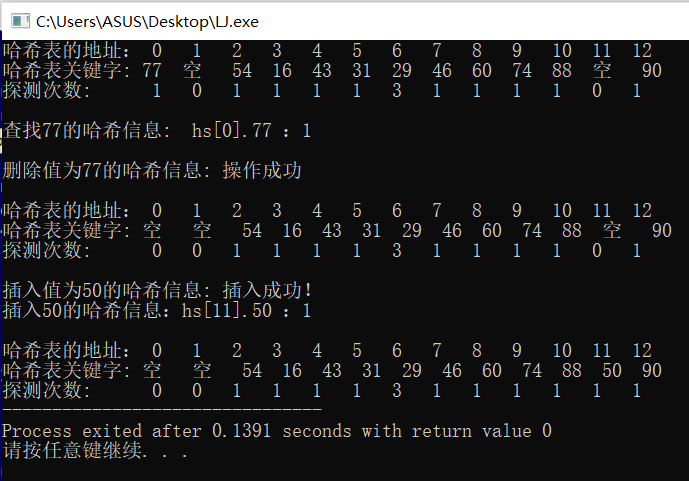展开全文• /* / / @哈希表(线性探测法) / */ #include<stdio.h> #include<stdlib.h> #include<windows.h> #define Field -1 #define null -32768 typedef struct hashmap...C语言
• 散列表（Hash table，也叫哈希表），是根据关键码值(Key value)而直接进行访问的数据结构。也就是说，它通过把关键码值映射到表中一个位置来访问记录，以加快查找的速度。这个映射函数叫做散列函数，存放记录的数组...二次探测
• 直接看代码： #include <stdio.h> #include <stdlib.h> //宏定义相关常量 #define Max 10 #define Size 10 typedef struct Sqlist{ int *data; int length;...//哈希表 int hash (int key){数据结构
• 哈希表是一种搜索结构，当数据量大时，哈希搜索的效率高，平均时间复杂度O(1)。 【哈希查找】： （1）在插入时，根据待插入元素的关键码，以此函数计算出该元素的存储位置并按此位置进行存放。 （2）在搜索时，对...
• 哈希表线性探测法和链地址法求查找成功与不成功的平均查找
• 散列表（Hash table，也叫哈希表），是依据关键码值(Key value)而直接进行訪问的数据结构。也就是说，它通过把关键码值映射到表中一个位置来訪问记录，以加快查找的速度。这个映射函数叫做散列函数，存放记录的数组...搜索 性能 哈希冲突
• 采用线性探测法作为冲突处理方法，将数据逐个放入大小为15的数组中。 输出散列表的存储示意图（第一行输出下标，第二行输出横线，第三行输出数据）。 并对其进行查找，输出（找到、找不到时的）查找次数。 2、输入10...数据结构 链表
• HashTable-散列表/哈希表，是根据关键字（key）而直接访问在内存存储位置的数据结构。 它通过一个关键值的函数将所需的数据映射到表中的位置来访问数据，这个映射函数叫做散列函数，存放记录的数组叫做散列表。哈希...
• 哈希表 将所有的数据，确定在某个范围内，对整体情况进行记录。 时间复杂度O(n2)->O(n) 如：在100个数据元素中查找各个数字出现的个数，数字范围为1~10 分析：100个整型数据，范围是1~10 首先，建立一个长度为10...数据结构 算法
• ## 哈希之线性探测法

千次阅读 2017-02-23 19:54:11
根据给定的一系列整数关键字和素数p,用除留余数法定义hash函数H(Key)=Key%p,将关键字映射到长度为p的哈希表中，用线性探测法解决冲突。重复关键字放在hash表中的同一位置。 Input 连续输入多组数据，每组输入数据...数据结构
• 今天我们主要的是用线性探测的方法处理哈希冲突的问题。 线性探测方法的具体实现如下： test.h #pragma once #include &lt;stdio.h&gt; #include &lt;stddef.h&gt; #include &lt;stdlib.h&...
• 一、哈希表 1、概念 哈希表(Hash Table)也叫散列表，是根据关键码值（Key Value）而直接进行访问的数据结构。它通过把关键码值映射到哈希表中的一个位置来访问记录，以加快查找的速度。这个映射函数就做散列函数，...数据结构
• 之前在写过的很多的结构...但是如果有一种存储结构，通过某种函数使元素的存储位置与他的关键码之间能够建立一一映射的关系，那么就能够直接地查找到需要地元素，时间复杂度就达到了O(1)，那么这种结构就是哈希表。...
• /** *哈希表： 优点：速度快（插入和查找） * 缺点：基于数组，不能有序遍历 * 键值对：通过键访问值 * 冲突：不同的关键字...开放地址线性探测 二次探测 再哈希法） * 2.链地址 */ public class Dat...
• 哈希概念 在之前学习过的顺序搜索和二叉树搜索中，元素存储位置和元素各关键码之间没有对应关系，因此在查找一个元素时，必须要经过关键码的多次比较。搜索的效率取决于搜索过程中元素的比较次数。 我们希望...
• 转自：...它通过把关键码值映射到哈希表中的一个位置来访问记录，以加快查找的速度。这个映射函数就做散列函数，存放记录的数组叫做散列表。2、散列存储的基本思路...
• 哈希表，是根据关键字（Key value）而直接访问在内存存储位置的数据结构。也就是说，它通过计算一个关于键值的函数，将所需查询的数据映射到表中一个位置来访问记录，这加快了查找速度。这个映射函数称做散列函数，...
• 分别画出用线性探测法和拉链法解决冲突时构造的哈希表，并求出在等概率情况下，这两种方法的查找成功和查找不成功的平均查找长度。 2.链地址法：用链地址发解决冲突的方法时：把所有关键字为同义词的记录...
• 6-4线性探测法的查找函数（20分） 试实现线性探测法的查找函数。 函数接口定义： Position Find( HashTable H, ElementType Key ); 其中HashTable是开放地址散列表，定义如下： #define MAXTABLESIZE ...
• ## 哈希表线性探测再散列的方法详解

万次阅读 多人点赞 2020-03-26 16:01:56
线性探测再散列的方法详解 例：设哈希表长为m=13，散列函数为H(k)=k mod 11，关键字序列为5，7，16，12，11，21，31，...试求：散列后的表中关键字分布（假定解决冲突的方法为线性探测再散列） 解题步骤： 1.将 ...数据结构
• } // 平方探测 Position Find(HashTable H, ElementType Key) { Position Currentpos, NewPos; int CNum = 0; // 记录冲突次数 // NewPos = Currentpos = Hash(Key, H->TableSize); // 初始散列的位置 int p = H->...
• 5.给定一个关键字序列（13，4，18，20，32，15，9，24），哈希表长度为 11，哈希函数为 H(Key)=Key%11，采用线性探测法解决冲突，画出构造的哈希表（8 分），并求出等概率查找时查找成功 的 ASL（成功） （1 分），...算法...